﻿ Failure Probability Assessment for Pipelines under the Corrosion Effect

### Failure Probability Assessment for Pipelines under the Corrosion EffectOPEN ACCESSPEER-REVIEWED

## Failure Probability Assessment for Pipelines under the Corrosion Effect

Mourad Nahal1,, Rabia Khelif1

1Department of Mechanical Engineering, Badji Mokhtar University, Annaba, Algeria

### Abstract

In this work, a numerical method was developed, by a reliability mechanical coupling, in order to define the reliability index and probability of failure evolutions for pipelines under corrosion effect. The chosen model, takes into account uniform and localized corrosion. Thus, the hardness and tensile tests were worked out to characterize the mechanical properties of pipelines material. Once the model was defined, a simulation was carried out by the software Phemica. The importance factors were also estimated. A methodology has been presented for the reliability analysis of pipelines subjected to localized corrosion. The variables influencing the reliability are treated as random variables and represented by suitable statistical distributions. An approximate limit state function was developed. Advanced first-order second moment reliability theory was employed for the estimation of the probability of pipeline failure by Phimeca software logiciel. From a numerical investigation, it was found that both defect depth and fluid pressure have significant influences on pipeline reliability.

### At a glance: Figures

1234
Prev Next

• Nahal, Mourad, and Rabia Khelif. "Failure Probability Assessment for Pipelines under the Corrosion Effect." American Journal of Mechanical Engineering 2.1 (2014): 15-20.
• Nahal, M. , & Khelif, R. (2014). Failure Probability Assessment for Pipelines under the Corrosion Effect. American Journal of Mechanical Engineering, 2(1), 15-20.
• Nahal, Mourad, and Rabia Khelif. "Failure Probability Assessment for Pipelines under the Corrosion Effect." American Journal of Mechanical Engineering 2, no. 1 (2014): 15-20.

 Import into BibTeX Import into EndNote Import into RefMan Import into RefWorks

### 1. Introduction

The exploit of pipelines is, to some level, the most efficient way to convey hydrocarbons. In Algeria, there are 11000 kilometers of gas and oil pipelines. Pipeline managers need assessments of integrity and safety in order to make appropriate decisions regarding the allocation of funds for maintenance and operation. Limited resources require rational and sound criteria for realistic assessment directed towards their best utilization. Over time, this assessment is resorting more to the use of structural reliability techniques [1, 2, 3, 4].

During service, the remaining strength of pipelines depends on number of factors, including the operational conditions, and defects introduced by construction, third-party damage, corrosion and ground movement, etc. In particular, corrosion and ground movement constitute two important causes resulting in pipeline failure [5, 6, 7, 8]. Corrosion is of concern because any loss of the pipe all thickness means a reduction of pipeline structural intensity and hence an increase in the risk of failure, while the ground movement produces a longitudinal load on the pipe, creating stress/strain to threaten the pipeline safety. Thus, a synergistic effect of corrosion and the soil-induced strain on the pipeline integrity should be considered in strain-based design of pipelines. Reliability methods are a powerful and useful tool when assessing defects in pipelines. The basis for a probabilistic assessment is the distributions of all variables, including load (internal pressure), material strength and the sizing accuracy of the inspection tool. There are many studies and papers on reliability based assessments of corroded pipelines, such as a computer program based on the FORM/SORM  procedure was developed to calculate failure probabilities when a crack-like defect size is obtained from nondestructive testing. Reliability analysis can be used for the assessment of pipeline safety. It can be used also as a rational and consistent tool for assessment of pipeline integrity when this is measured in terms of containment. The analysis can also be used to assess the effect of the various random parameters on the estimate of pipeline safety. This could help pipeline operators make proper decisions about maintenance, repair and replacement programs. The present analysis is confined to strength assessment of pressurized pipelines subject to transport hydrocarbons under high pressure. It is assumed that these flaws mainly interrupt the load carrying capacity of pipelines in direct proportion to their depths.

### 2. Corrosion Model

The geometry of corrosion is usually described by uniform corrosion or located corrosion, the majority of problems of corrosion affecting the structures are a combination of these two forms. The distribution of corrosion in pipes can be stochastically described by the space random fields. Consequently all the depth of corrosion at any place x and time t can be described by the sum of these two types of corrosion: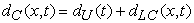(1)

where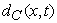is the total corrosion depth at the location x and time t,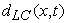is the depth of the localized corrosion defect and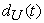is the depth of the uniform corrosion. Figure 1 shows a longitudinally-oriented surface corrosion defect in the wall of a pressurized pipeline. In this figure,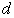is the pipe wall thickness,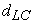is the depth of localized corrosion,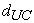is the depth of uniform corrosion and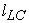is the length of the corroded region projected on the longitudinal axis.

2.1. Uniform Corrosion

A practical engineering way to account for uniform corrosion is to use a power law to model the loss of wall thickness with the exposure time [8, 11]. The general form of the corrosion power law is written as: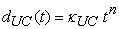(2)

where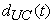is the thickness of the corroded layer, t is the elapsed time (i.e. age of the pipe) and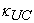and n are the corrosion constants, to be evaluated by fitting Eq. (2) from field corrosion data [5- 6]. For atmospheric pressure, the mean and standard deviation are, respectively, 0.066 and 0.037 for the multiplier, and 0.53 and 0.14 for the power n .

2.2. Localized Corrosion

In the past decades, extensive researches have been performed on localized corrosion, in order to derive empirical models for different environmental conditions. The mostly used empirical equations for the time-dependent corrosion depth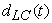and length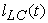take the form [6, 9]: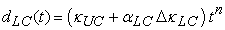(3)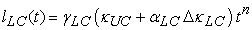(4)

whereandare respectively the localized corrosion depth and length,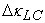is the specific rate of localized corrosion,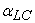is the localized corrosion fraction and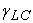is the length-to-depth ratio of localized corrosion.

2.3. Failure Pressure

The defects form a region of stress concentration, thus interrupting the normal hoop force trajectories along its length, depth and compelling the interrupted tensile hoop force to be redistributed around it, like is showing in Figure 1. The primary failure mechanism is generally considered to be the extension of the defect through the remaining portion of the pipe wall. At failure, the pipe wall is severed and the failure occurs either by leak or by rupture. The type of failure is dependent on the size of the resulting through-wall defect; attention is confined herein only to the failure event, not to the type of failure.

An expression largely used in order to estimate the constraint of failure given in the following form: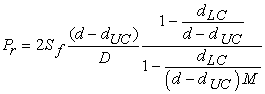(5)

where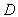is the pipe diameter,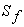is the flow stress, defined by multiplying the yield stress by a factor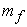: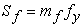. Ahammed and Melchers  proposed to modelby a lognormal distribution with mean value equal to 1.1 and coefficient of variation of 0.05.

2.4. Folias Factor

A comparative study of Folias factors was developed in literature , where the correlation between the Folias factor M and L²/ (Dt) is plotted which is given in Figure 2. M1, M2, M3 and M4 represent the expressions of Folias factor in NG-18, B31G and PCORRC respectively. The K in the Figure 2 represents the value of L²/ (Dt). It can be seen from Figure 2 that the Folias factor in the B31G is larger than that in the NG-18 in spite of their same form about corroded pipe assessment criterion. The form of PCORRC is different than NG-18 and B31G its Folias factor cannot be directly compared to these two .

The Folias factor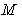(also known as bulging factor) is a semi-empirical factor that covers the fracture mechanics aspects; it is given by :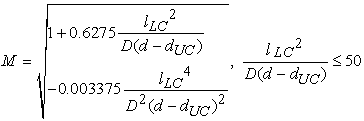(6)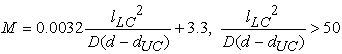(7)

### 3. Reliability Analysis

The concept of reliability being posed, a criterion of failure is defined using a function known as a performance (or limit state), noted G, and which depends on the random variables of the model. The analysis of reliability enables us to know the criticality of failures which can arrive at structures (pipeline), as well as the economic impact of these failures, and hence provides mandatory information for inspection planning. So, it is necessary to define a limit state function.

The reliability R of a structure is conventionally defined in the following way [3, 4]: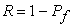(8)

Where the probability of failure Pf corresponds to the probability of occurrence of the event G ({X}) < 0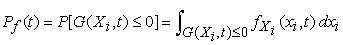(9)

The limit state function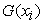corresponds to the security border, which is conventionally defined by the difference between the pipe pressure resistanceand the applied pressure P: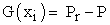(10)

where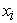are the realizations of the random variables of the pipe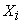. This margin is defined such that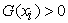indicates safety and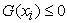corresponds to conventional failure. So, by replacing Pr by P we obtained: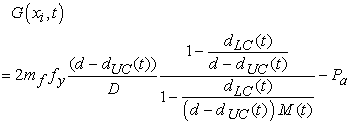(11)(12)

where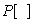is the probability operator and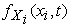is the joint probability density function of the random variables at time t. As the limit state function is nonlinear in terms of the input random variables, iterative algorithms  are conveniently applied in order to reduce the computation time. In the present work, the First Order Reliability Method, known as FORM , is applied to compute the reliability index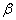, which is defined as the minimum distance between the median point and the failure domain in the standard Gaussian space. This index is evaluated by solving iteratively the constrained optimization problem: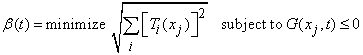(13)

where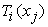is the probabilistic transformation of the model variables to standard Gaussian variables. The solution of this optimization problem can be obtained by optimization or reliability algorithms [11, 12, 13]. The failure probability can thus be evaluated by the first order approximation: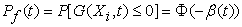(14)

where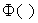is the cumulative Gaussian probability function.

### 4. Pipeline Reliability Assessment

To evaluate reliability or probability of failure, we are applied to use a software simulation (Phémica). A summary of the input data used in the probabilistic corrosion assessment is given in Table 1 and more details on the input assumptions are given below. Also, the acceptable defect size can be estimated based on the acceptable failure probability. In order to illustrate the above concepts, a pipeline with known corrosion defects was analyzed. This reflects the situation where defect dimensions are known from measurements by ‘intelligent’ tools. The random variables considered in this study are presented in Table 2 along with the typical statistical values and distribution functions for the variables.

#### Table 2. Data for an example pipeline reliability analysis

4.1. Experimental Study

The workshop TSS (seamless tube), which was conducted the study, carried out a number of quality tests on its production. In the case of tubes for transport of hydrocarbons, the objective it was obtaining the resistance Rp0,5 (strain conventional), Rm (maximum strength).

A elongation and hardness HRC, during tensile, elongation and hardness tests. The machine used for these tests is a tensile testing machine Zwick / Roell equipped with a sensor and hydraulic jaws-loaded from the specimen through maintaining heels together at both ends (Figure 4).

Tensile test: The test specimens used, according to the U.S. standard API. The goal is to determine the maximum strength and strain conventional, and we obtained the following results: RP0.5 = 302.7 MPa and Rm = 470.5 MPa.

4.2. Experimental Results

The reliability index calculation was carried out using Monte Carlo simulation which is integrate on PHEMECA data , and 106 simulation times is applied. The calculation results are shown in the Figure 7, the figure show a rather fast reduction in the index of reliability opposite the lifespan of pipeline, influenced by the various constraints which the structure undergoes.

Figure 8 shows the probability variation of failure depending on the defect depth generated by the corrosion phenomenon for four cases, and we see that there is a proportional relationship between the probability of failure and defect depth. In addition when the defect depth is important, we can see an important increase of the probability of failure. So the defect depth affects aggressively the structure studied.

In this figure we show a second case, by the change in the probability of failure as a function of the defect length is presented. And we can conclude that here also the variation has a proportional relationship with the defect length caused by corrosion. Four cases were taken, we can notice that whenever we increase the defect length can be seen a significant increase in the probability of failure.

The two curves showed in Figure 10 and Figure 11 show the variation in the reliability index as a function of both defect depth and defect length variation. Where we can say that there is a clear decrease in the reliability index when the defect becomes more important.

### 5. Assessment Reliability Index with Different Folias Factor

In this section of work, we determined the reliability index using different Folias factor which are given respectively by, NG-18, B31G and PCORRC. The found result is shown in the following curve. The curve show a difference between three reliability indexes, where we can see a better result of the one who has a relationship with B31G.

The above figure shows the variation of sensitivity factors according to the corrosion depth. The idea is to divide the corrosion depth at different percentages thickness of pipe, after that we see sensitivity factors evolution. The found result gives a domination of two essential factors, the operating pressure and the maximum strength of material, considering the influence of corrosion weakens this resistance and increases the impact of the operating pressure which generates a significant constraint more in the internal month of the pipe walls.

### 6. Conclusion

This study was conducted for a goal to predict and evaluate the parameters of pipeline safety, which undergo to corrosion phenomenon, which is considered as a major problem that affects the tubes intended for the carriage of hydrocarbons. The probability of failure and reliability index, were calculated using probabilistic models based on the reliability-mechanical coupling. A comparative study of the evolution of the reliability index based on various Folias factors was also made, which aims to choose the best result, where we found a better evolution of reliability that takes into account the Folias factor given by B31G.

A methodology has been presented for the reliability analysis of pipelines subjected to localized corrosion. The variables influencing the reliability are treated as random variables and represented by suitable statistical distributions. An approximate limit state function was developed. Advanced first-order second moment reliability theory was employed for the estimation of the probability of pipeline failure by Phimeca software. From a numerical investigation, it was found that both defect depth and fluid pressure have significant influences on pipeline reliability.

### Acknowledgment

The authors are grateful to Arcelor piping and tubes, Company for the material and mechanical testing.

### References

  M.D. PandeyProbabilistic models for condition assessment of oil and gas pipelines. NDT&E International, Vol. 31, No. 5, pp. 349-358, 1998.In article CrossRef  Cronin Duane S, Pick Roy J. Prediction of the failure pressure for complex corrosion defects. Int J Pressure Vessels Piping 2002; 79: 279-287.In article CrossRef  Ping Hong H. Inspection and maintenance planning of pipeline under external corrosion considering generation of new defects. Struct Saf 1999; 21:203-222.In article CrossRef  Kurt R, Leis B, Cox D, Pan J. Probabilistic analysis of piping systems. The Fourth National Congress on Pressure Vessel and Piping Technology: Random Fatigue Life Prediction. Portland, Oregon 1983.In article  Muhlbaucer WK. Pipeline risk management manual. In: Idea, techniques and resources. 3rd ed. Elsveier; 2004.In article  Han Ping Hong. Inspection and maintenance planning of pipeline under external corrosion considering generation of new defects. Structural Safety 21 (1999) 203-222.In article CrossRef  Parkins RN. A review of stress corrosion cracking of high-pressure gas pipelines. In: Corrosion’2000, paper no. 363. Houston: NACE; 2000.In article  Baker M. Stress corrosion cracking studies, integrity management program DTRS56-02-D-70036. Department of Transportation, Ofﬁce and Pipeline Safety; 2004.In article  Kiefner, J. F. & Vieth, P. H., PC program speeds new criterion for evaluating corroded pipe, Oil Gas J., 20 (1990) 91-3.In article  Hopkins, P. & Jones, D. G., A study of the behavior of long and complex-shaped corrosion in transmission pipelines. In Proc. 11th Int. Con5 on Offshore Mechanics and Arctic Engineering, Vol. V, Part A, ASME 1992, pp. 211-17.In article  Bubenik, T. A., Olson, R. J., Stephens, D. R. & Francini, R. B., Analyzing the pressure strength of corroded pipeline. In Proc. 11th Int. Conf. on Offshore Mechanics and Arctic Engineering. Vol. V, Part A, ASME 1992, pp. 225-31.In article  Melchers, R. E., Structural Reliability: Analysis and Prediction. Ellis Horwood, Chichester, UK, 1987.In article  PHIMECA Engineering. PHIMECA—reliability-based design and analysis. User’s manual, version 1.6, Aubière, France; 2002.In article  G. Zhang et al. Research on probabilistic assessment method based on the corroded pipeline assessment criteria / International Journal of Pressure Vessels and Piping xxx (2012) 1-6.In article CrossRef  ASME-B31G. Manual for determining the Remaining Strength of Corroded Pipelines. A Supplement to ASME B31 Code for Pressure Piping. American Society for Mechanical Engineers, New York, 1991.In article  M.Nahal&R.Khelif. Mechanical reliability analysis of tubes intended for hydrocarbons. Journal of Mechanical Science and Technology February 2013, Volume 27, Issue 2, pp 367-374.In article CrossRef